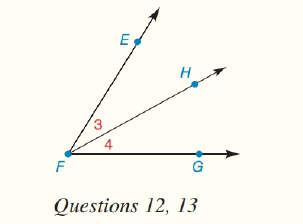Chapter 1.CT, Problem 13CT### Elementary Geometry for College St...

6th Edition
Daniel C. Alexander + 1 other
ISBN: 9781285195698

#### Solutions

Chapter
Section### Elementary Geometry for College St...

6th Edition
Daniel C. Alexander + 1 other
ISBN: 9781285195698
Textbook Problem
1 views

# In the figure, m ∠ 3 = x and m ∠ 4 = 2 x − 3 . If m ∠ E F G = 69 ° , find: a) x___________ b) m ∠ 4 ________To determine

(a)

To find:

The value of x.

Explanation

Given:

m3=x, m4=2x3 and mEFG=69°.

The given figure is shown below.

Approach:

According to Angle-Addition postulate, if point B lies in the interior of AOC then, mAOB+mBOC=mAOC

To determine

(b)

To find:

The value of m4.

### Still sussing out bartleby?

Check out a sample textbook solution.

See a sample solution

#### The Solution to Your Study Problems

Bartleby provides explanations to thousands of textbook problems written by our experts, many with advanced degrees!

Get Started